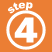Home    |    Teacher    |    Parents    |    Glossary    |    About UsMatch each statement with an inequality. Inequality The sum of a number and five more than the number is less than fifteen. a. n + 7 > 15 A number times five is greater than fifteen. b. n + 9 < 30 A number less negative seven is less than or equal to five. c. n - 7 < 0 The difference between a number and seven is less than zero. d. 5n > 15 The sum of a number and seven is greater than or equal to fifteen. e. n - (-7) < 5 A number is greater than fifteen. f. 2n < 20 Twice a number is less than twenty. g. n > 15 The sum of a number and nine is less than thirty. h. n + (n + 5) < 15
 Homework Help | Algebra | The Language of AlgebraEmail this page to a friendSearch·  Definitions·  Order of Operations·  Writing equations·  Writing inequalitiesFirst Glance In Depth Examples WorkoutWriting inequalities